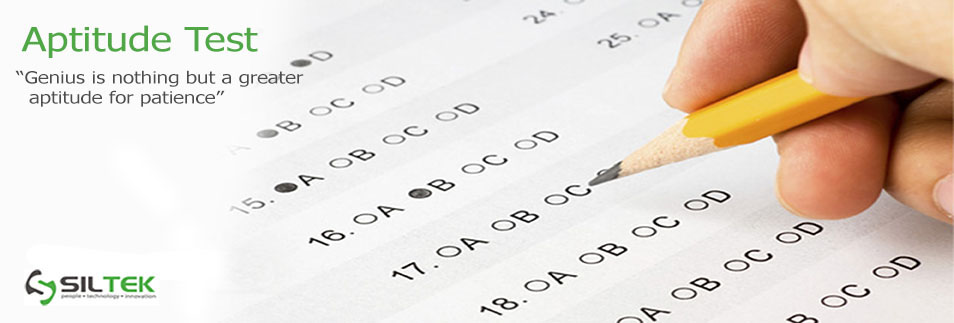An Aptitude test is a written evaluation of a candidate's basic skills of Quantitative Aptitude, Logic, Reasoning and English.

Quantitative Aptitude

It is a test of one's reasoning ability on mathematical skills and the comprehension of mathematical concepts. This section contains questions on Number Systems, Arithmetic, Geometry, Algebra etc. This section may also include elucidation of a given graphic or tabular format.

Logical Reasoning

This tests the competence of a student to deduce information from the given data. The data may include various mind stimulators such as puzzles, riddles, syllogisms (deductive logic), critical reasoning (inductive logic) etc.